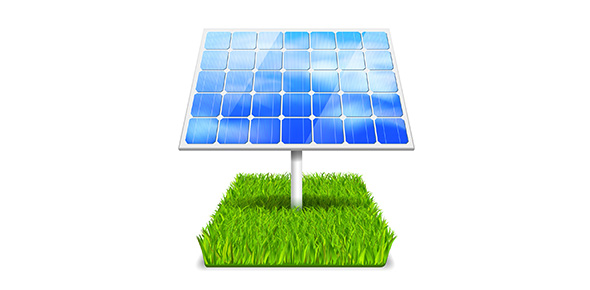# How Much Do You Know About Geothermal Energy? Trivia Quiz

13 Questions | Attempts: 3894
ShareSettingsHow much do you know about geothermal energy? It is one of the natural sources of energy and it is found within the subsurface of the earth. Power plants use the heat to generate steam to make electricity though advance this type of energy has been used by human kind for too long. What are some of the dangers to accessing this type of energy? This quiz will help you learn some more about it.

• 1.
What are the two main types of energy?
• A.

Chemical and thermal

• B.

Electrical and nuclear

• C.

Potential and kinetic

• D.

None of the above

• 2.
The amount of kinetic energy depends on two things, the mass and _________________ of an object.
• A.

Weight

• B.

Acceleration

• C.

Energy

• D.

Velocity

• 3.
A type of potential energy that is associated with objects that can be stretched or compressed is called:
• A.

Mechanical energy

• B.

Elastic potential energy

• C.

Gravitational potential energy

• D.

None of the above

• 4.
A car moving, a frog leaping, and sounds that are made are all examples of what kind of energy?
• A.

Chemical

• B.

Electrical

• C.

Thermal

• D.

Mechanical

• 5.
A type of potential energy that depends on height and is equal to the work done to lift the object is called:
• A.

Vertical energy

• B.

Gravitational potential energy

• C.

Elastic potential energy

• D.

Growing energy

• 6.
Which of the following are good examples of elastic potential energy?
• A.

Rubber band

• B.

Bow

• C.

Bungee cord

• D.

All of the above

• 7.
What is the formula used to calculate kinetic energy?
• A.

KE = mass x velocity squared divided by 2

• B.

KE = mass x work divided by 2

• C.

KE = force x mass divided by 2

• D.

None of the above

• 8.
Visible light, ultraviolet radiation and microwaves are all good examples of:
• A.

Electrical energy

• B.

Nuclear energy

• C.

Electromagnetic energy

• D.

None of the above

• 9.
When a nucleus splits apart it is called:
• A.

Nuclear fission

• B.

Nuclear fusion

• C.

Nuclear energy

• D.

None of the above

• 10.
Static shock, batteries and powerlines all are good examples of what kind of energy?
• A.

Electrical

• B.

Electromagnetic

• C.

Nuclear

• D.

None of the above

• 11.
Mechanical energy can be potential or kinetic.
• A.

True

• B.

False

• 12.
The total energy of the particles in an object is thermal energy.
• A.

True

• B.

False

• 13.
Potential energy is the energy of motion and kinetic energy is stored.
• A.

True

• B.

False

## Related TopicsBack to top
×

Wait!
Here's an interesting quiz for you.